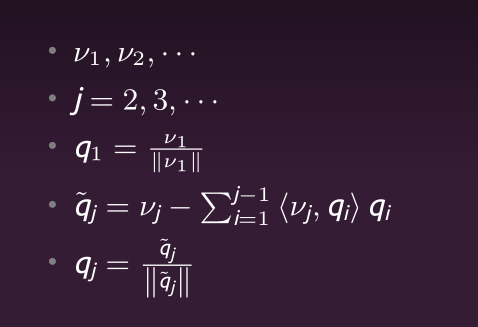【数值计算】花式解线性方程组

Posted by Cww97 on 2017-09-27

* Assignment1 LU分解 * codes * 参考文献
* Assignment2 迭代法 * Problem * 数据生成 * norm * slow_Jacobi * 清真_Jacobi * Gauss-Seidel * SOR超松弛法 * 参考文献
* Assignment3CGQR * Problem * Conjugate Gradient Method 共轭梯度法 * QR Method * 参考文献

python 矩阵操作

Assignment2: 迭代法

TAGS: 数值计算

vector norm

matrix norm

slow_Jacobi

Wiki为啥他能跑这么快Xk+1=D−1(b−Rxk)

Gauss-Seidel

xk+1=BGxk+fG

BG=(D−L)−1，fG=(D−L)−1b

SOR超松弛法

xk+1=Bwxk+fw

Bw=(D−wL)−1[(1−w)D+wU]

fw=w(D−wL)−1b

output:

参考文献

 Lecture-3课程ppt

 wiki_jacobi

 数值计算——线性方程组的迭代法

 胡 枫 ,于福溪 《超松弛迭代法中松弛因子 ω的选取方法》 青海师范大学学报 2006.01.13 42-46

 互动百科_松弛法

 numpy文档_上三角矩阵

 numpy文档_norm

Assignment3：CG&QR

2017/10/19

Problem

Calculate the equation Ax = b through Conjugate Gradient Method
and QR Method respectively

Conjugate Gradient Method 共轭梯度法

wiki，中文wiki只有寥寥几句，英文的比较详细

1. 先x，用到了上一步的x和p，
2. r,用到上一步的r和p
3. p，用到刚刚算出的r和上一步的p 很显然可以发现，x，r，p只需要一个变量就可以完成循环

QR Method（tips：这里的R特别容易算错）

参考文献

 dot常见error

 numpy线性代数

 numpy行列操作